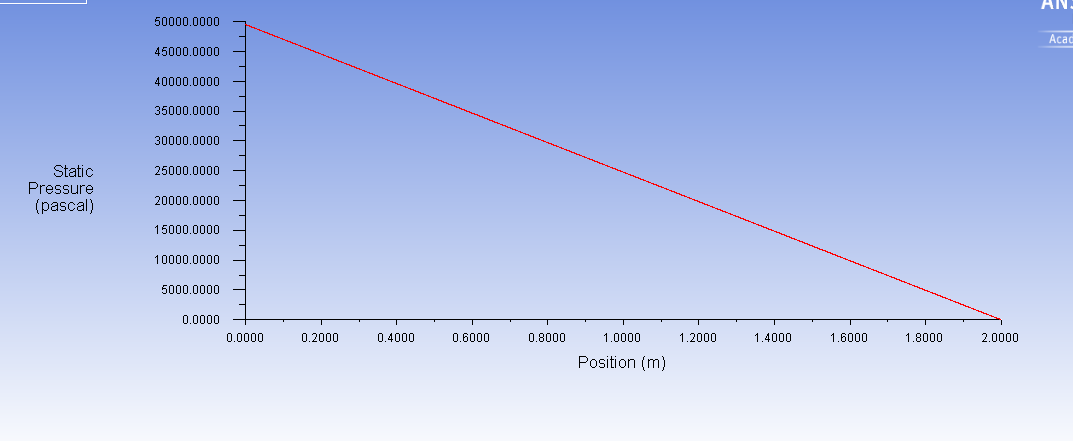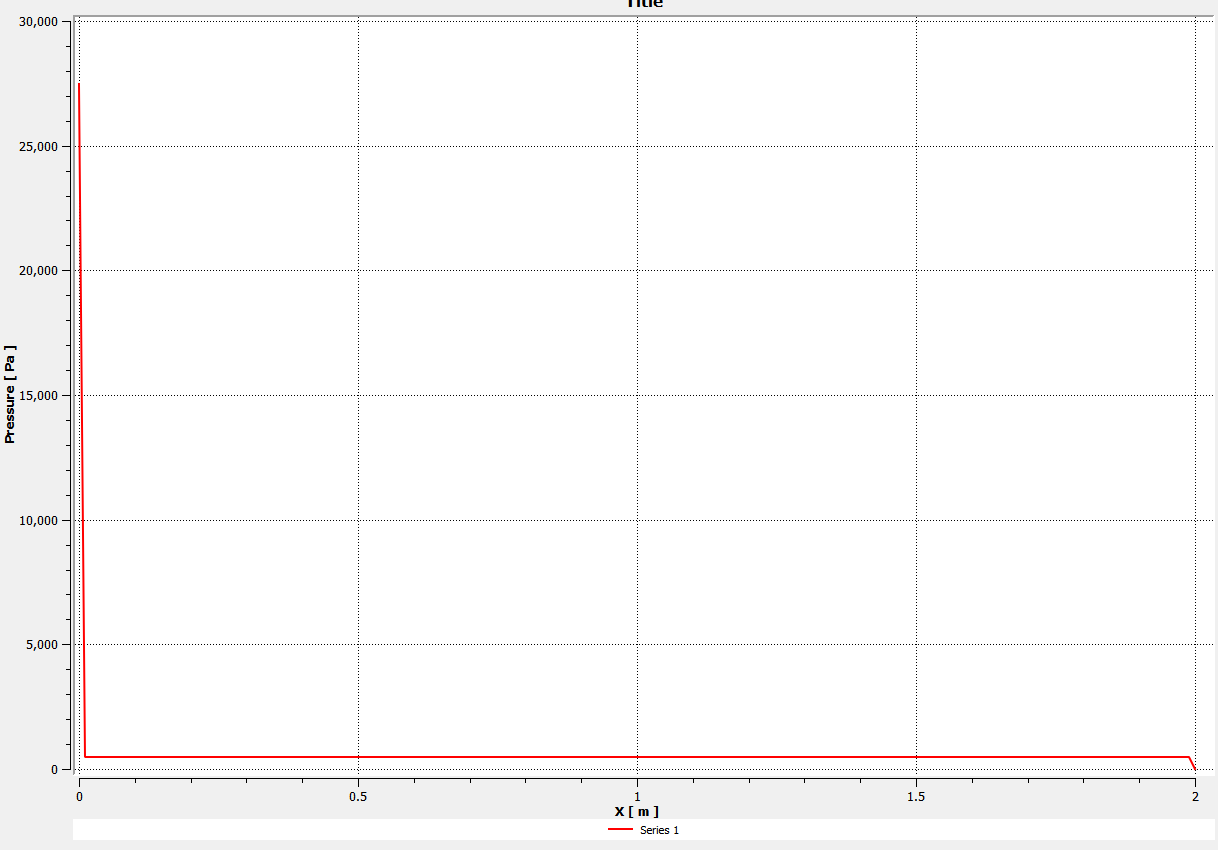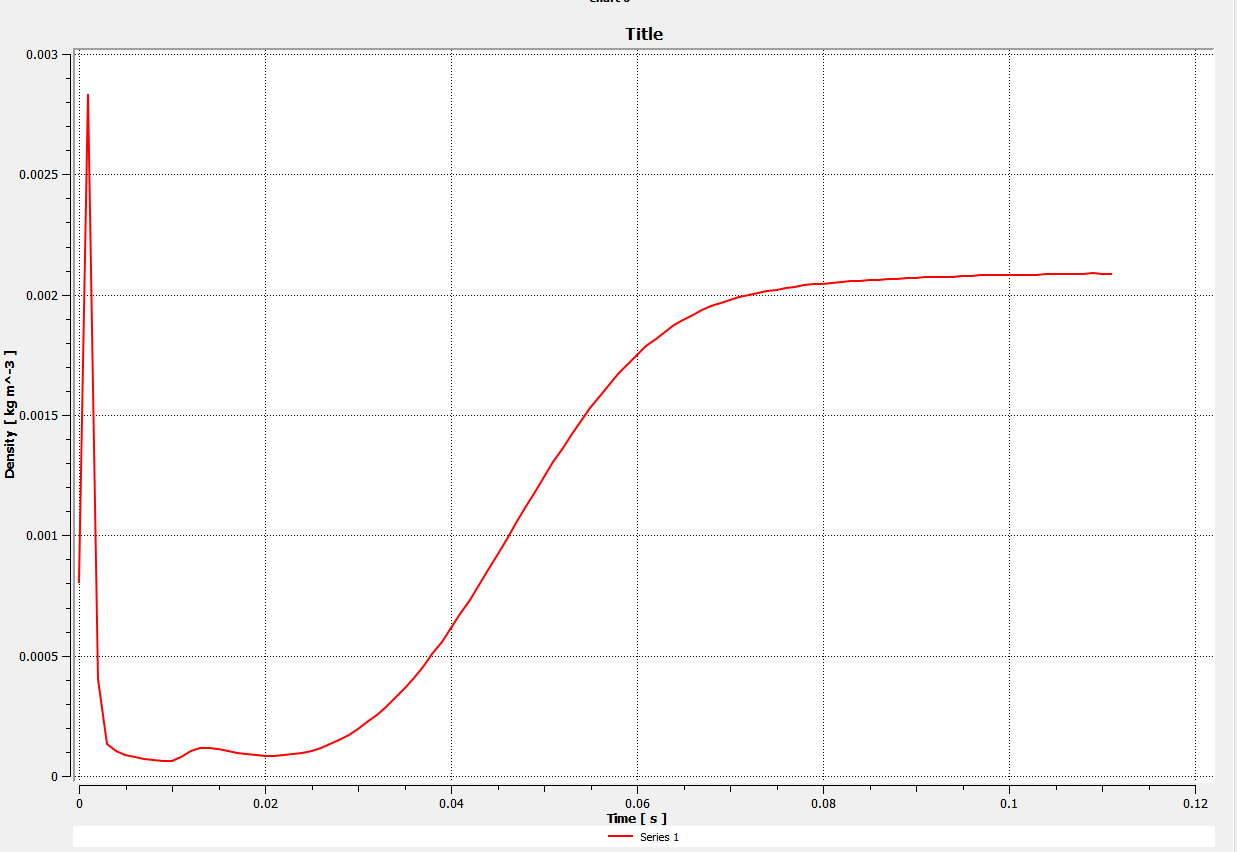## Fluids

#### finite values inside the simulation domain with hybrid initialization at t =0

•sgbaek
Subscriber

I had a similar question below (Transient compressible pipe flow into vacuum) , and I would like to refine my issue regarding the hybrid initialization. I am modeling a nozzle compressible flow into a vacuum with a pressure inlet and outlet boundaries. One thing I found with the hybrid initialization is that it assigns finite pressure, velocity, and density inside the simulation domain. The figure below shows the initial pressure profile after the hybrid initialization. One can see that ANSYS assigned the pressure down the pipe.

However, since I am interested in the propagation of the flow, I do not think this was a correct initialization. I kind of expected to have zero pressure within the simulation domain, and I could see the flow propagation down the pipe to the chamber. I patched the internal region with the zero pressure, but the solution diverged even at the 1st step.

My question is whether this hybrid initialization is a proper way to examine the arrival time of the gas at a some distance away from the inlet boundary? This delayed rise at a given location and the time it takes to reach the steady state are my two goal from this simulation.

Thanks,

John

•Vishal Ganore
Ansys Employee

Do standard initialization with 0 values at time t=0. Then you could see how flow is getting propagated in a flow domain during iterations.

•sgbaek
Subscriber

Dear Vishal

Since I have another similar posting below, I am going to consolidate them in this post. Thanks for going over my issue. I will try your suggestion of doing a standard initialization with 0 values of pressure and velocity, instead of the hybrid initialization. Meanwhile here is my update, and my question is at the end of this post. Based on Raef's reply that was suggesting an initialization of the field variables, I tried to use the "patch" function. My model geometry is now a 2 m long pipe with a 1 mm diameter. I modeled a 2d axisymmetric case. I am assigning a pressure-inlet of 50000 Pa and the pressure outlet of 0 Pa at the other hand. The flow near the end of the pipe becomes choked, so the problem is still compressible fluid.

The pressure and density profiles at the t=0 step after a hybrid initialization:So I patched a 500 torr pressure value on the entire internal zone, using a patch function, although ideally I wanted to patch the zero pressure everywhere (this is related to my question below). The below shows the pressure field as a function of the pipe location at the t=0 step. You can see a delta function like pressure field only at the inlet.I was able to advance the time steps, and, at x=2m, I am getting the following density evolution as a function of time:one can see a nice build-up of the density as a function of the time, except the peak near at t ~ 0 sec. I think the peak at around t ~ 0 sec is due to the finite pressure (500 torr).

My question: When I used the patch function to set the pressure of the entire zone to zero in order to remove the peak near at t ~0 sec, the solver gave me an error message of divergence. The minimum low pressure I could assign in the pipe was ~ 110 Pa. Below this, the solver did not like it. What could be a reason for this? And how this patching would be different from a setting a zero pressure in a standard initialization? (I imagine that both result in a same pressure field, but I will definitely try your suggestion of using a standard initialization with zero pressures.)

Sincerely,

John